## Friday, March 29, 2013

### HP 39gii Programming Tutorial - Part 5: Pixels and Graphics

Welcome everyone to Part 5 of my HP 39gii programming tutorial.

We are going to work with some graphic commands with the HP 39gii.

The Screen

When it comes to graphics, the HP 39gii has two systems: points and pixels.

Points refers to Cartesian points. Points work just as you would expect: x increases as you go right, y increases as you go up. The boundary of the screen are controlled by the value of Xmin, Xmax, Ymin, and Ymax.

Pixels refer to the LCD terminal screen. Unless you are working with the Plot screen, you are most likely going to work with the LCD terminal screen. In the pixel system, each coordinate is fixed. The 39gii screen is 255 pixels by 126 pixels. In the pixel system, the upper left hand coordinate is (0,0), and the bottom right hand corner is (254,125). The x coordinate increases as you go to the right, but the y coordinate increases as you go down. A row of (about) 14 pixels is reserved for the soft key menus (F1 - F6). In practice you would deal a screen of size 255 × 111.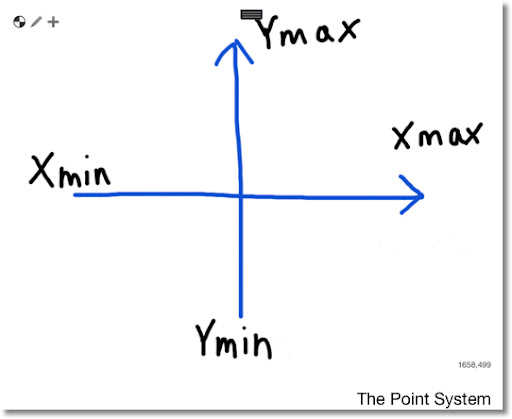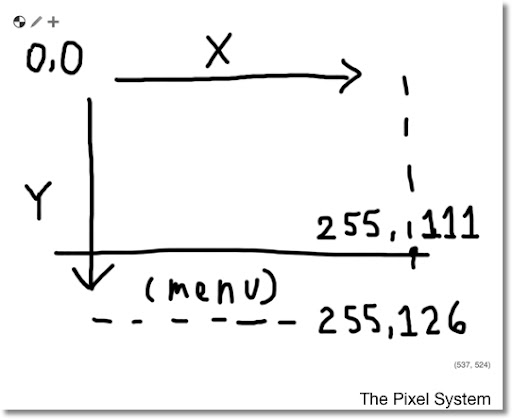We will work with the Pixel system in this part of the tutorial.

Common Drawing Commands

In a program, you will want to erase the drawing screen, and at the end, you will need a "hold" command.

Erasing the screen is pretty simple. Whenever you want to erase the LCD screen, type RECT();.

The "hold" command freezes the screen together to show you the picture. Without the "hold" command the calculator just returns to the Home screen. Two "hold" commands are WAIT(0) and FREEZE. I prefer WAIT(0) because the program continues with the press of any key and it works as expected.

Some of the common drawing commands are:

Erase the drawing screen:

RECT();

Key Sequence:
[SHIFT] [Math] [ F1 ] [ 4 ] [ ) ]

To put text anywhere on the screen, starting from pixel (x,y):

TEXTOUT_P(string,x,y);

Key Sequence:
[SHIFT] [Math] [ F1 ] [ 4 ] [ up ] [ENTER]

To draw a line segment from pixel (x1, y1) to (x2, y2):

LINE_P(x1,y1,x2,y2);

Key Sequence:
[SHIFT] [Math] [ F1 ] [ 4 ] [ LN ]

To draw a white box with black border, given opposite corner pixels (x1, y1) and (x2, y2):

RECT_P(x1,y1,x2,y2,0,3);

Key Sequence:
[SHIFT] [Math] [ F1 ] [ 4 ] [ ÷ ]

To draw a circle with center pixel (x,y) and radius of r pixels:

ARC_P(x,y,r);

Key Sequence:
[SHIFT] [Math] [ F1 ] [ 4 ] [ 2 ]

To draw an arc between angle A and B:

ARC_P(x,y,r,A,B);

Key Sequence:
[SHIFT] [Math] [ F1 ] [ 4 ] [ 2 ]

To turn the pixel (x,y) on:

PIXON_P(x,y);

Key Sequence:
[SHIFT] [Math] [ F1 ] [ 4 ] [ ( ]

To turn the pixel (x,y) off:

PIXOFF_P(x,y);

Key Sequence:
[SHIFT] [Math] [ F1 ] [ 4 ] [ x² ]

Colors (Really Grayscale) on the 39gii

The 39gii has four grayscale shades. Each have the following values:
0 = black
1 = dark gray
2 = light gray
3 = white

You can use color in the following commands. The color arguments are always optional.

RECT_P(x1,y1,x2,y2,edge color,fill color);
LINE_P(x1,y1,x2,y2,color);
PIXON_P(x,y,color);
ARC_P(x,y,r,A,B,color);

Fonts with the 39gii

The HP 39gii has two fonts: large and small. They have the following values:
0 (or left out) = default, whatever the system setting is
1 = force small font
2 = force large font

The syntax of TEXTOUT_P with font size is:
TEXTOUT_P(string,x,y,font size);

Let's use these commands in some programs.

The Program RTEXT

RTEXT(desired string, number of places)

RTEXT places a desired string all over the screen at random.

EXPORT RTEXT(str1,times)
LOCAL X,Y,K;
RECT();
RANDSEED(RANDOM);
FOR K FROM 1 TO times DO
X:=INT(RANDOM(1,254));
Y:=INT(RANDOM(1,111));
TEXTOUT_P(str1,X,Y);
END;
WAIT(0);
END;

Example: Sprinkle "39gii" in 75 random places. From the Home screen type RTEXT("39gii",75). You get something like this: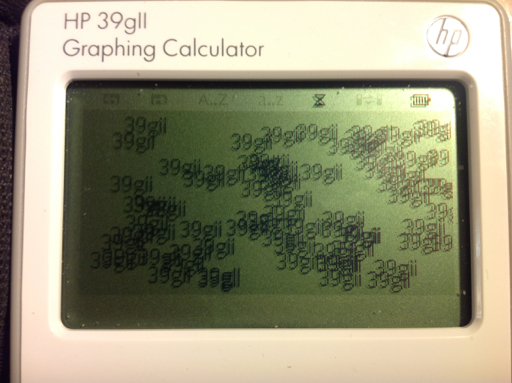The Program BOXLH

The program BOXLH draws a box of length L and height H. The box grows from the center pixel (127, 56). Input: BOXLH(L,H)

EXPORT BOXLH(L,H)
BEGIN
RECT();
RECT_P(127-L/2,56-H/2,127+L/2,56+H/2,0,3);
WAIT(0);
END;

Example:
Draw a box with length of 60 pixels and height of 56 pixels. From the home screen type BOX(60,56).The Program BOX3D

BOX3D takes the box drawing program one step further, by adding some depth to make the box look three-dimensional.

Input: BOX3D(L,H,D,A)
L = length of the box in pixels
H = height of the box in pixels
D = depth of the box in pixels
A = tilt angle in degrees

BOX3D sets the angle mode to Degrees. (HAngle = 0).

EXPORT BOX3D(L,H,D,A)
BEGIN
LOCAL X,Y,Z,T,C,S;
HAngle:=1;
RECT();
X:=127-L/2;
Y:=127+L/2;
Z:=56-H/2;
T:=56+H/2;
RECT_P(X,Z,Y,T,0,3);
C:=D*SIN(A);
S:=D*COS(A);
RECT_P(X+C,Z-S,Y+C,T-S,0,3);
LINE_P(X,Z,X+C,Z-S);
LINE_P(Y,Z,X+C,Z-S);
LINE_P(X,T,X+C,T-S);
LINE_P(Y,T,Y+C,T-S);
WAIT(0);
END;

Note: The order of the drawing commands is important, especially when it comes to the RECT and RECT_P commands.

Example: Length of 45 pixels, height of 27 pixels, depth of 33 pixels, at an angle of 45°
Enter BOX3D(45,27,33,45)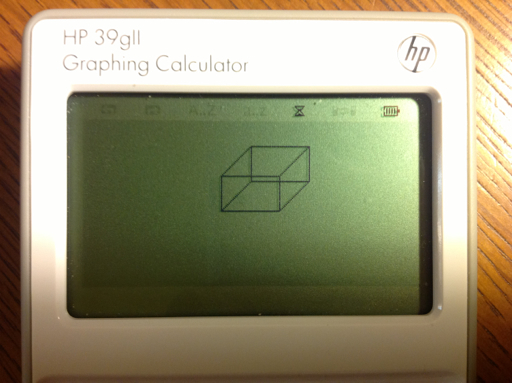A Short Animation: The Program GROWTH

GROWTH is a program of an arc that grows by π/6 (30°) every second until a circle is completed. Radians angle mode (HAngle=1) is set in this program.

EXPORT GROWTH()
BEGIN
RECT();
HAngle:=0;
FOR K FROM 0 TO 2π STEP π/6 DO
ARC_P(127,56,30,0,K);
WAIT(1);
END;
TEXTOUT_P("Done",0,0);
WAIT(0);
END;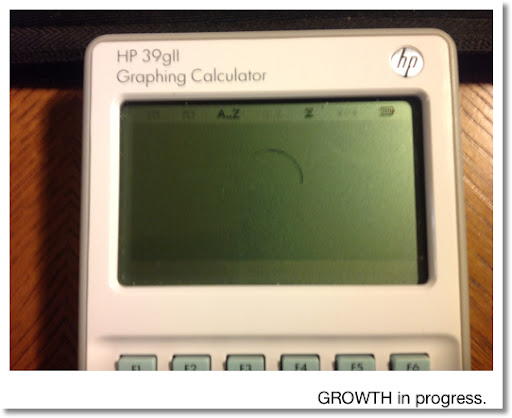CDEMO: Colors and Fonts

CDEMO shows the three colors and the small font. You can cut down on the lines needed by using a clever mathematical equation and a well set up FOR loop.

EXPORT CDEMO()
BEGIN
LOCAL K;
RECT();
FOR K FROM 1 TO 3 DO
RECT_P(100,30*K,200,30*K+25,0,K);
END;
TEXTOUT_P("Dark Gray",1,35,1);
TEXTOUT_P("Light Gray",1,65,1);
TEXTOUT_P("White",1,95,1);
TEXTOUT_P("39gII Colors",50,1,2);
WAIT(0);
END;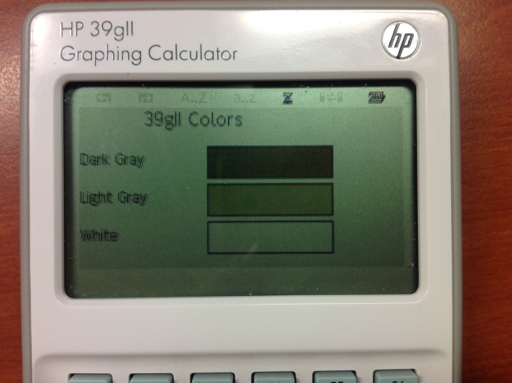Well, I hope these five programs demonstrate what you can do with the graphing commands with the 39gii. As always, I hope encourages you to program and to try things out!

In Part 6, we are going interactive!

Until next time,

Eddie

This blog is property of Edward Shore. 2013